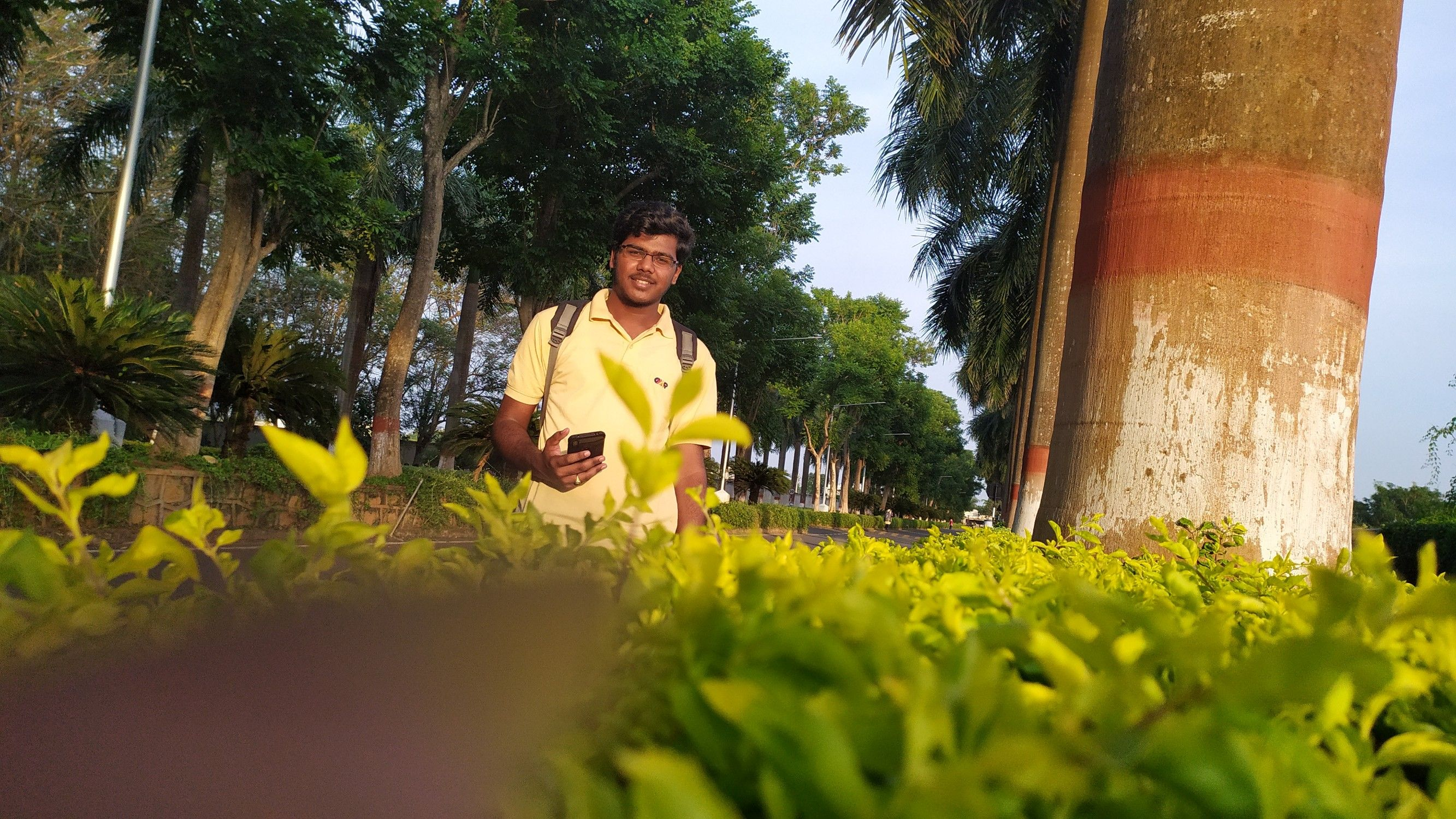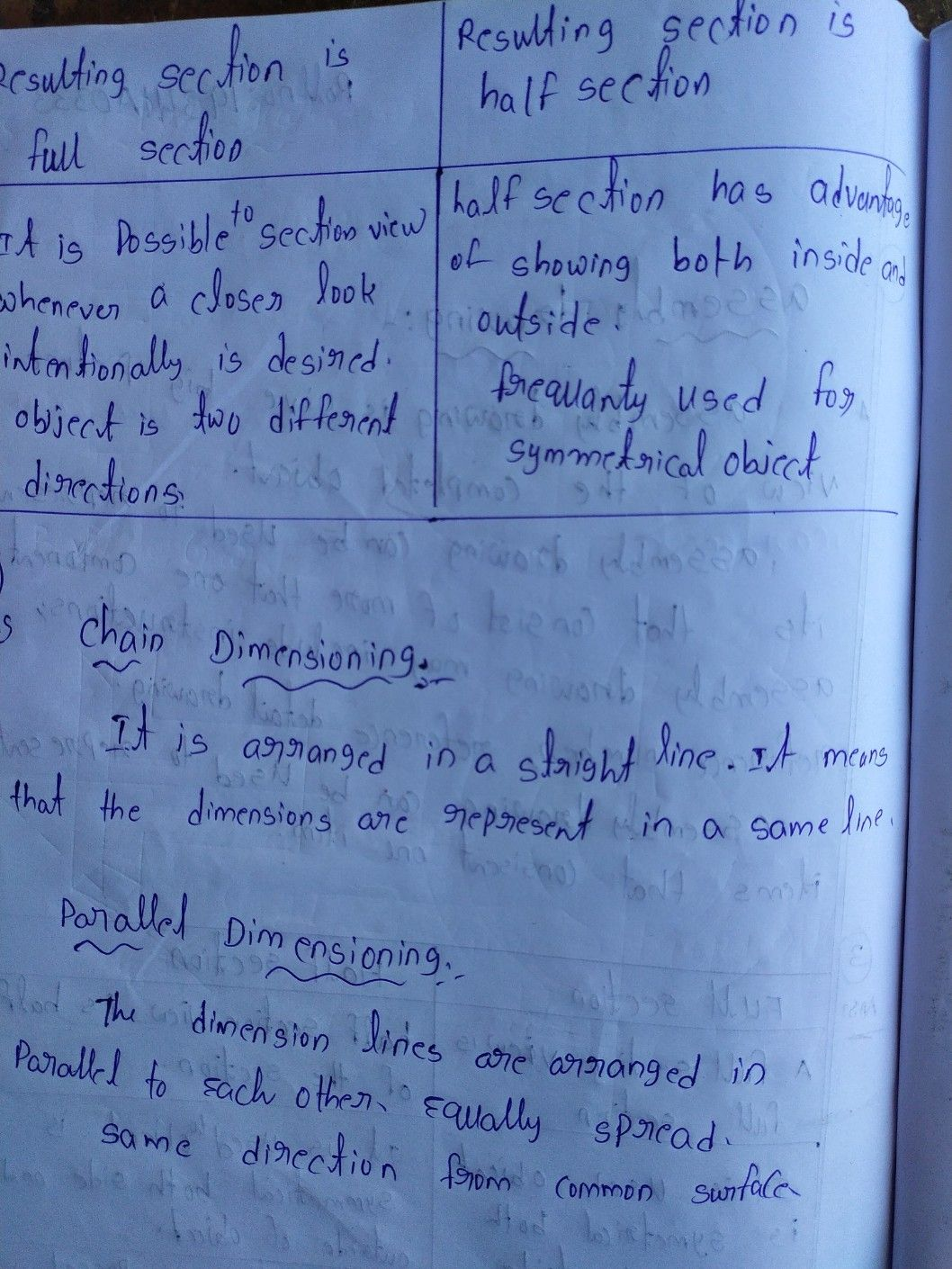Symbol
ProblemA. ARITHMETIC SEQUENCE Solve the following $r0blcm\right)s$ 1.The arithmetic mean between two terms in an arithmetic sequence $s39.1fon$ of these terms is 32, find the other term. 2. If five arithmetic means are inserted $oct0yccn=9$ and 9, what is the third 3. What are the first and last terms of an arithmetic sequence when its $rdmcan7$ $anthmetic$ means are 35, 15 and -5? 4. Find the value of x if the arithmetic mean $of3and3x+5is8$ 5. Find the value of a when the arithmetic mean $ofa+7anda+3is3a+$ +9. GEOMETRIC SEQUENCE B. Solve the following $0r0blcms$ 15,.....? 1. What is the 5 term of the geometric sequence $e3\sqrt{20,3/2,15}$ 2. Find the sixth term of a geometric sequence where the second term is 6 and the common ratio is 2. 3. The geometric mean between the first two terms in a geometric sequence is 32. If the third term is 4, find the first term, 4. Three positive numbers form a geometric sequence. If the geometric mean of the first two numbers is 6 and the geometric mean of the last two numbers is 24, find the three numbers and their common ratio. 5. Find the sum of the terms of a geometric sequence where the first term is 4, the last term is 324 and the common ratio is 3. C. DIVISION OF POLYNOMIALS Solve the following $pr0blcms$ 1. The side of a square $otis\left(5x-3\right)n$ meters. How many meters of fencing materials are needed to enclose the square lot? If one square meter of the lot costs Ph 15,000, what is the cost of the square lot? 2. A $ectangula1$ garden in a backyard has an area $of\left(3x^{2}+5x-6\right)$ square meters. Its width is (x $+2\right)metcrs$ a. Find the length of the garden b. You $dccidc$ to partition the garden into two or more smaller congruent $2ardcns$ Design a $p0ssib|$ $0$ model and include mathematical concepts in your design. 3. $1fr=2x^{3}+4x^{2}-x-6a$ and $s=x-2$ What is r/s ?